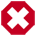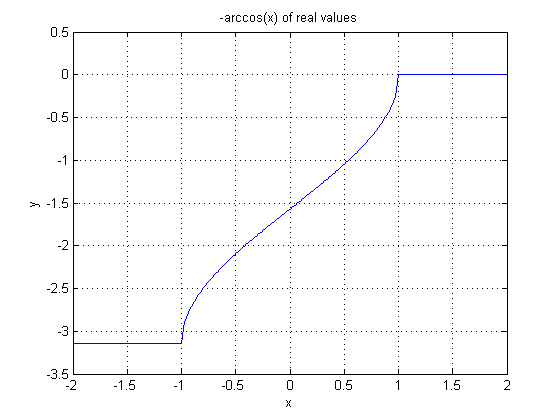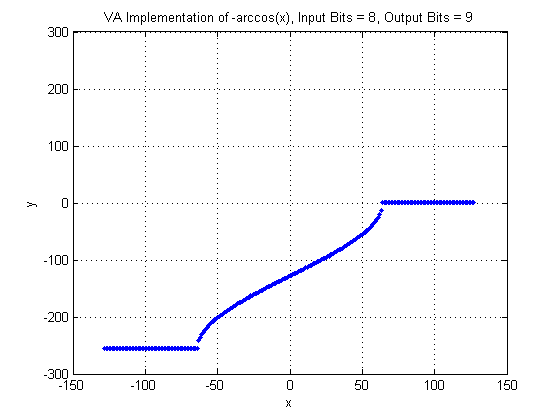## Operator ARCCOS

Implemented Function ist not arccos(x)The implemented function is -1 * arccos(x). See description.

The operator ARCCOS is calculating the arc cosine of the input. Note that not arccos(x) is implemented. Instead, the result is inverted i.e. -1 * arccos(x) is implemented.

The input range of arccos is [-1,1]. The operator maps the input interval range [-1, 1] to

where

is the bit width at the input. As can be seen, not the full value range of the input is used. If an input value is outside the allowed value range, the operator will clip the value to -1 or 1. Thus, the argument x of the arccos is determined by

The operator calculates the inverse of the arccos i.e. the output value range is not [0, - π] it is [- π, 0]. The output value range of the operator in VisualApplets is mapped to

where

is the bit width at the output link. Thus the output value is

The following image shows the plot of the normal inverse arccos function.In the next figure, the VisualApplets operator implementation is shown. Note the input and output bit widths.### I/O Properties

Property Value
Operator Type O
Input Link I, data input
Output Link O, data output

### Supported Link Format

Bit Width [8, 12] [8,32]
Arithmetic signed signed
Parallelism any as I
Kernel Columns 1 as I
Kernel Rows 1 as I
Img Protocol {VALT_IMAGE2D, VALT_LINE1D, VALT_PIXEL0D} as I
Color Format VAF_GRAY as I
Color Flavor FL_NONE as I
Max. Img Width any as I
Max. Img Height any as I

None